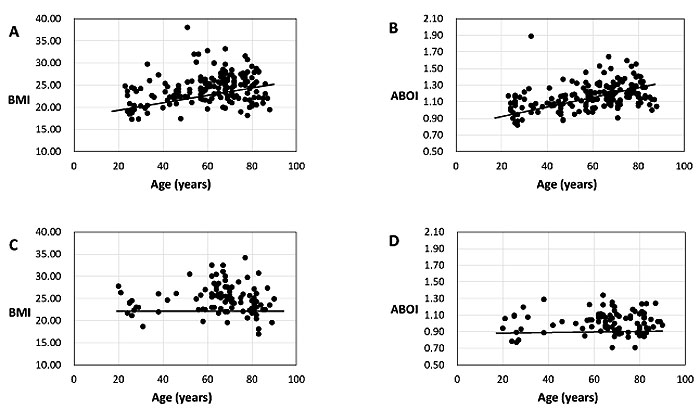Fig. (2) BMI and ABOI of female and male subjects of different ages. The ages of female subjects in this study were 23-88 years and the ages of male subjects 20-90 years. A) Female BMI vs age with mean BMI of 24.14 and a standard deviation of 3.35 (r2 = 0.106, p < 0.001). B) Female ABOI vs age with mean ABOI of 1.17 and a standard deviation of 0.16 (r2 = 0.162, p < 0.001). C) Male BMI vs age with mean BMI of 24.86 and a standard deviation of 3.25 (r2 = 0.0001, p < 0.726). D) Male ABOI vs age with mean ABOI of 1.01 and a standard deviation of 0.13 (r2 = 0.003, p = 0.670).